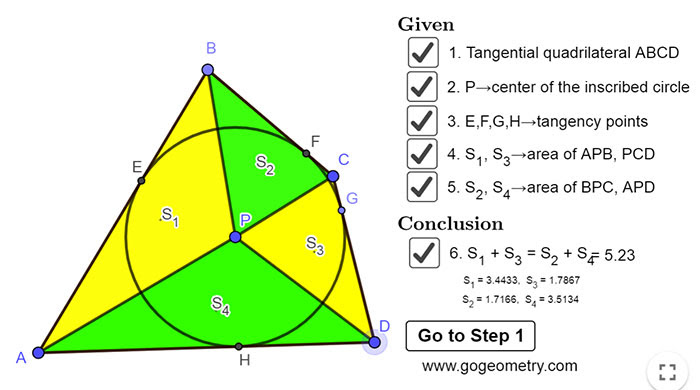## Monday, March 23, 2020

### Geometry Problem 1465: Tangential Quadrilateral, Incenter, Inscribed Circle, Equal Sum of Areas

Interactive step-by-step animation using GeoGebra. Post your solution in the comment box below.
Level: Mathematics Education, High School, Honors Geometry, College.

Details: Click on the figure below.1.P1465
AE=AH, BE=BF, CG=FC, DG=DH => AE+BE+CG+DG=AH+BF+FC+DH =>
(AE+BE+CG+DG).R =(AH+BF+FC+DH).R
S yellow = S green

2.By tangent property, AH=AE, BE=BF, CF=CG, DG=DH

On the other hand, the height of those triangles are the same, namely the radius,
AD*r/2 + BC*r/2 = AB*r/2 + CD*r/2
Hence, S1+S3 = S2+S4.

3.We have 4 pairs of congruent Tr.s, (APH,APE), (BPE,BPF), (CPF,CPG), (DPH,DPG) one each of which is yellow and the other green.

The result is thus easily derived

Sumith Peiris
Moratuwa
Sri Lanka